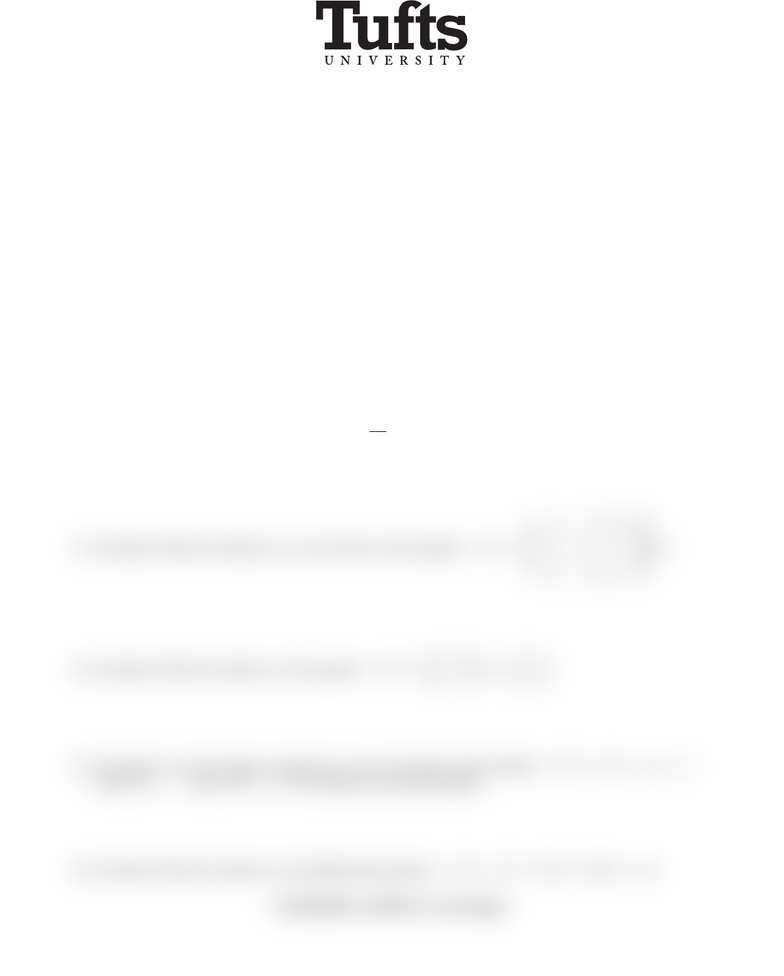Study Guides (400,000)
US (230,000)
Tufts (400)
MATH (200)
All (30)
Final

# MATH51 PastExams Final-S13Exam

This preview shows half of the first page. to view the full 2 pages of the document.Mathematics 51 Differential Equations
Examination III May 6, 2013, 8:30–10:30am
No calculators, books or notes are allowed on the exam. All electronic devices must be turned off
and put away.You must show all your work in the blue book in order to receive full credit.
Make sure to sign your blue
book. With your signature you are pledging that you have neither given nor received assistance on
the exam.
Good luck!
1. (10 points)
a. Show that x(t) = t+ 4 is a solution of the differential equation sin(t)D3x+4Dxx=t.
b. Write sin(t)D3x+ 4Dx x=tas a system of differential equations.
c . Give
one
solution to the system
in vector form
.
2. (10 points) Solve the initial-value problem dx
dt =x2,x(0) = 3.
3. (10 points) Find all solutions (
in vector form
) to the equation D~x =
5 3 0 0
31 0 0
0 0 1 0
0 0 0 7
~x.
4. (10 points) Find all solutions to the equation D~x =3 0
0 5 ~x +1
et
5. (10 points)
Use the Laplace transform
to solve the initial value problem D2x+Dx 2x= 2
with x(0) = 1and x(0) = 0. No credit by any other method.
6. (10 points) Find all solutions to the differential equation ((D1)2+ 1)(D+ 3)Dx = 12.
Examination continues on next page